Introduction :
We were interested in the development of computing tools and the operation of direct and inverse geometric models (DGM and IGM) for a planar 3R robot.  Simulations and the modeling of its movement were done for its path planning.
Primary language: Matlab.

Testing DGM and IGM :
Simulations :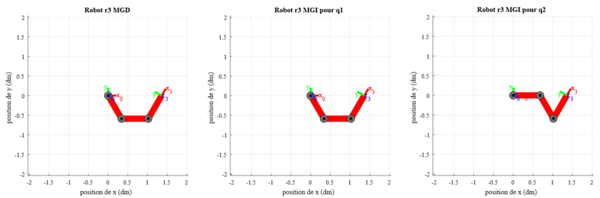Planar 3R Robot :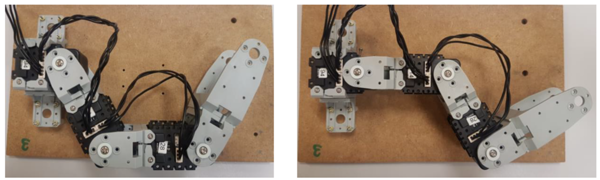Trajectory Planning :
In Articular Space :
The planar 3R robot makes a movement, between 2 points (initial point and final point) via a sequence of desired intermediate points, in order to avoid obstacles. The trajectory is free between theses intermediate points. So, the generation of movement can be done directly in the configuration space: it is translated by a sequence of articular positions constituting the instructions of the servo controllers.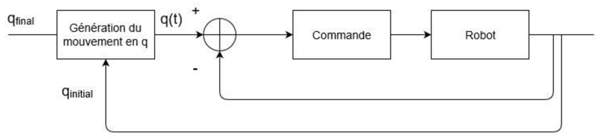In Operational Space :

The planar 3R robot makes a movement between 2 points, it being constrained between the points (e.g. rectilinear trajectory), or a movement between 2 points via intermediate points, the trajectory being constrained between the intermediate points. In these cases, since the trajectory is fixed at all moments in the operational space, it is preferable to reason in this space. The generated control law must then be converted into articular instructions by the coordinate loader.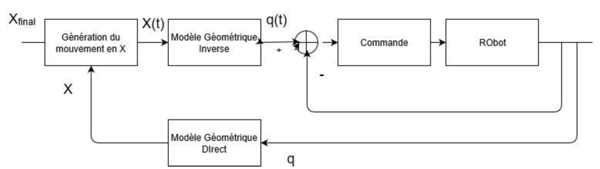Writing a Single Letter ('M') :

For the task of writing a letter with the planar 3R robot, it is necessary to easily control the final position thus the position of the terminal organ. Thus, we generate its movement in the operational space.

Simulations :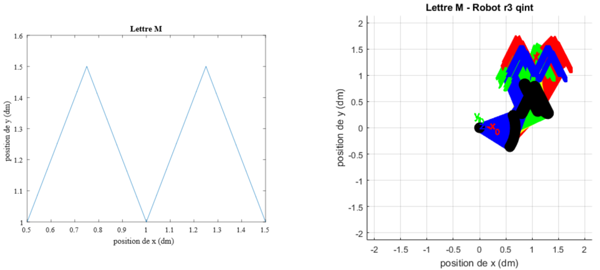Planar 3R Robot :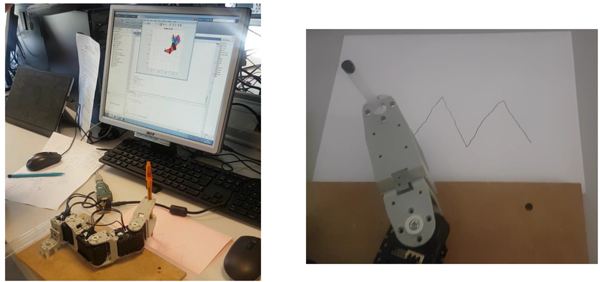Matlab Implementation :
GitHub Code